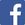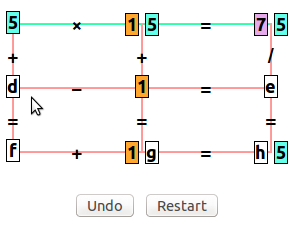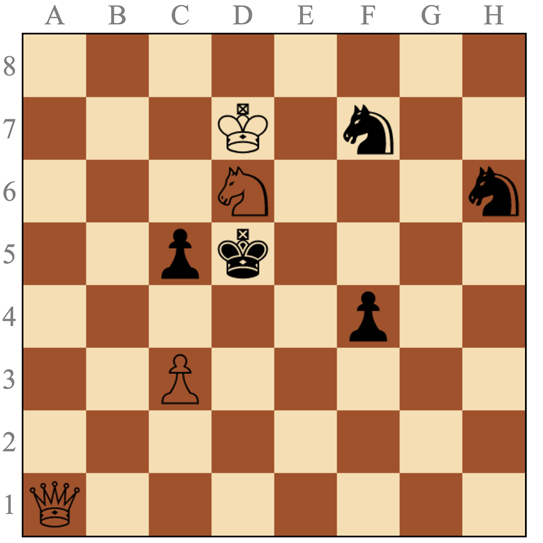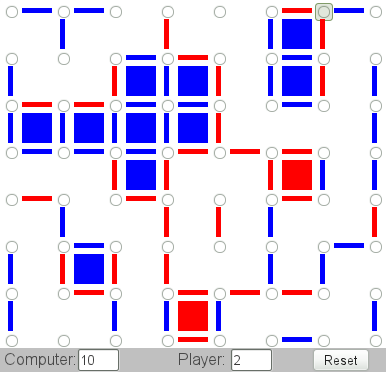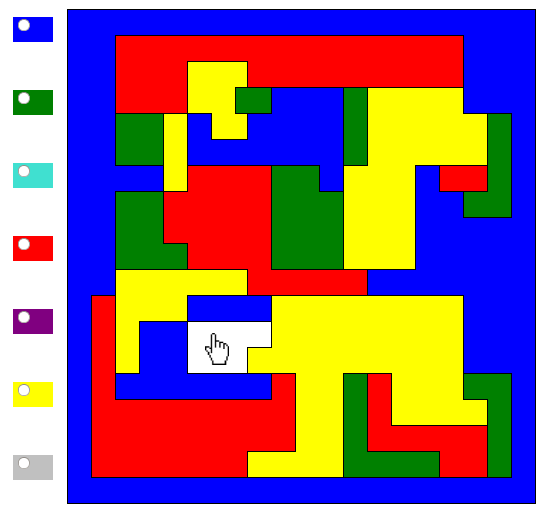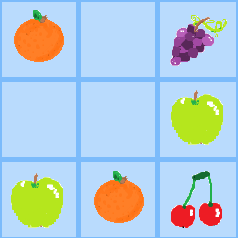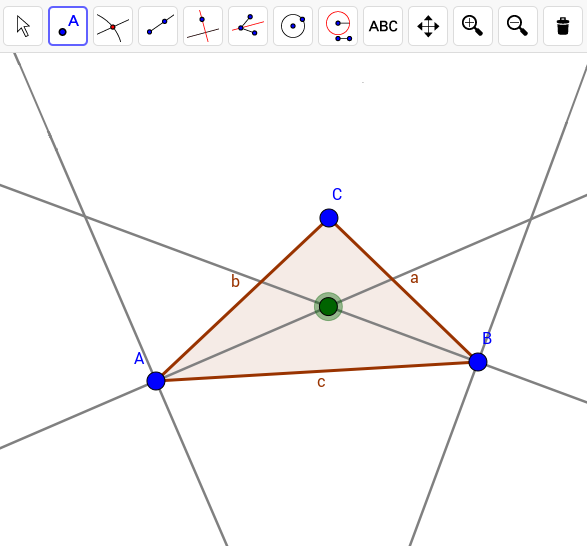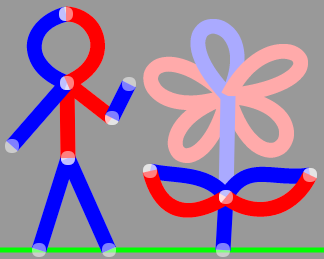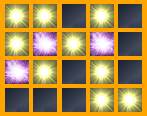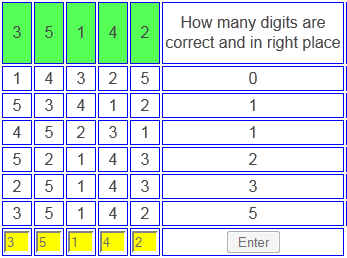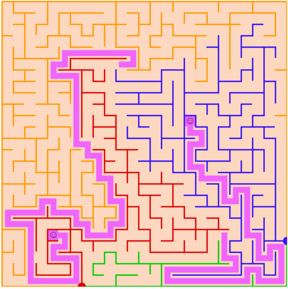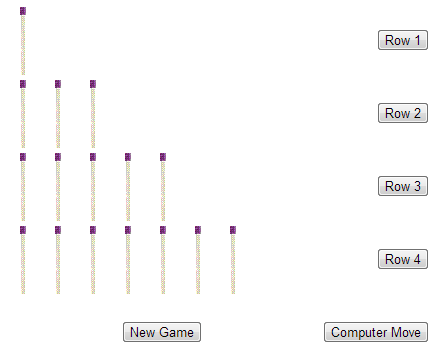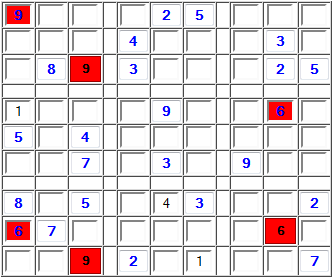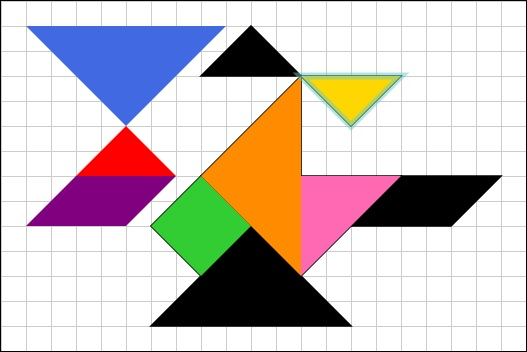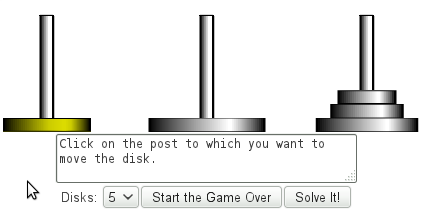## Today's Calcrostic Puzzle

``` ab - cd =  ec
+ ×  × ×   +
fg ×  h = jgd
= =  = =   =
jcg + de = cjb
```
What is This? | How to Solve It?

## Yesterday's Solution

```60 ×  4 = 240
- ×  × /   +
45 × 16 = 720
= =  = =   =
15 × 64 = 960
```
Total number of plays of all games: 10,650,014